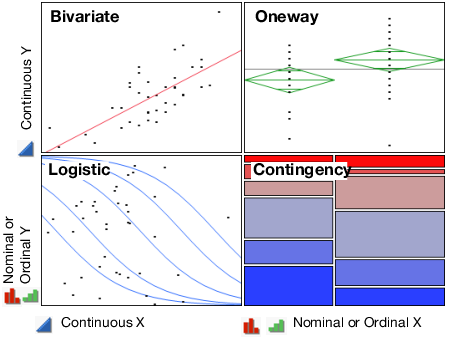Basic Analysis > Introduction to Fit Y by X
Publication date: 11/10/2021

# Introduction to Fit Y by X

##### Examine Relationships between Two Variables

Use the Fit Y by X platform to analyze pairs of variables. You can do this using scatter plots, linear regression, ANOVA, multiple comparisons, logistic regression, contingency tables and much more. Specific analyses depend on the modeling types of the variables.

Fit Y by X launches one of four platforms:

Use Bivariate to analyze a continuous Y and a continuous X

Use One-way analysis, including ANOVA, to analyze a continuous Y and a categorical X

Use Logistic regression to analyze a categorical Y and a continuous X

Use Contingency to analyze a categorical Y and a categorical X

Figure 4.1 Examples of Four Types of Analyses